# Spinout / The Brain / Chinese Rings puzzle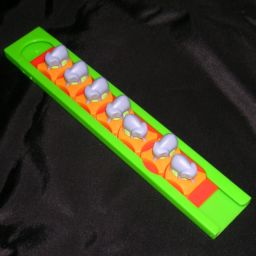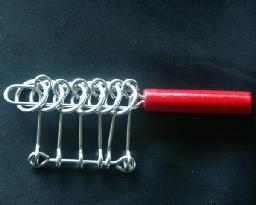Spinout by Binary Arts (now called Think Fun), is a simple puzzle consisting of a long flat plastic rectangle inside a grey cover. The piece could simply slide out if it were not for 7 oddly shaped dials. If all the dials are turned horizontal it will slide, but a dial can only be turned if the one to its right is vertical and any others further to the right are horizontal. This puzzle is now sold as Elephant Spinout, with a new design where the dials have little elephant figures on them, and a nice release mechanism so you can reset the dials.
There is an interesting design flaw in the Spinout that allows for an unintended shorter solution. The smaller versions of Spinout made in Taiwan and which have dials of different colours, have an especially short alternative solution.

Spinout was invented and patented by William Keister (US 3,637,215, filed 22 December 1970, granted 25 January 1972). This is the fourth puzzle Binary Arts made, and the first successful one. Their first puzzle was the Hexadecimal Puzzle, also by Keister.

The Brain, by Mag-Nif, consists of a tower of eight transparent disks that can rotate about their centres. The disks have slots in them and there are eight upright black rods going through the stack. The rods have two positions, in or out, and the aim is to move all the rods out. The rods are numbered 1 to 8, and a rod can only move if the rod with the next lower number is out and the further lower rods are in.

The Chinese rings puzzle is a row of rings, each affixed to a base with a rod. Each ring encloses the rod of the next ring in the row. A long loop of wire goes through all the rings and around all the rods. A ring can only be released from or placed back on the loop if the next ring is on the loop but all other rings further down are already off.

If your browser supports it, you can click on the link below to see a Javascript version of the Spinout puzzle.

## Solution:

All three puzzles have the same basic solution, which is based on the (standard) binary Gray code. In essence a binary Gray code is a list of all binary numbers of a certain length in such an order that any two adjacent numbers in the list differ only by one digit. This obviously corresponds to the puzzles, where each dial/rod/ring has two positions and you can only move one at a time.

All these puzzles are quite simple. In any position there are at most two possible moves, the equivalent of going up or down the list of Gray code numbers. If you never take back a move, you will always go on until you reach the end/beginning of the list. Unfortunately the actual puzzle might only be solved at the other end of the list, and you will have to go all the way back.

With the Chinese Rings and Spinout you have two choices for your first move, either ring/dial 1 or 2. If there are an even number of rings/dials, then you must begin with the even ring/dial i.e. number 2, if there are an odd number of rings/dials (the standard spinout has 7) then begin with the odd one i.e. number 1.

If you begin with the wrong move, you end with the position where all but the last ring/dial is off the loop/horizontal. You must then go all the way back to the beginning, and then do twice as many moves again to solve the puzzle. Note that with Spinout, there is a shortcut solution you can use in this situation (see below).

With the Brain you only have one first move, so it is easy to solve. Once in the solved position there are two choices for the next move if you want to bring it back to the initial position. Since there are an even number of rods, your next move then must be rod 2.

Suppose you find one of these puzzle in an intermediate position, and want to determine which of the two moves you need to solve it from there. The way to deduce this is best shown by a few examples.

Suppose the rods 1-8 of the Brain are In/Out/Out/In/Out/Out/In/In. If you want to solve it, then you would want the most difficult rod to move, rod 8, to be moved out. To do this, you must have rod 7 in and 1-6 out. Rods 5-7 are already in that position, but 4 is not so that has to be moved first. To move rod 4 you must have rod 3 in and 1-2 out, so rod 3 must be moved first. Therefore we need rod 2 in and rod 1 out. To move rod 2 in, we must have rod 1 in. This is already the case, so we can move rod 2 to get closer to the solution.

A similar argument would work if we wanted to get back to the starting position, all rods in. In that case we must move rod 6 in; to do that we need rod 5 in first; for that we need 4 in and 1-3 out; hence we must move rod 1.

On spinout we could make the same kind of argument. The dials are numbered 1-7 where dial 1 is the one that can always be moved, . Being right-handed I have dial 1 on the right, so in the starting position all the dials point upwards. Suppose they are all horizontal except 2,5,7. To move 7 horizontal we need 6 to be vertical. To move 6 we need 5 vertical and 1-4 horizontal. Therefore we need to move 2 horizontal and to do that 1 must be moved vertical first.

This argument also shows why we must turn dial 1 first in the starting position where all 7 dials are vertical. Dial 7 must be moved horizontal, so we need 6 vertical and 5-1 horizontal. To get 5 horizontal we first must get 4 vertical and 3-1 horizontal. In order to get 3 horizontal we must have 2 vertical and 1 horizontal. Therefore dial 1 must be moved first.

Solution Length:
The length of the solution (for any sized puzzle) can be easily calculated by recursion. Let Ln be the solution length for a puzzle with n dials/pins/rings. Clearly L1=1 and L2=2. To solve a puzzle with n pieces, first we have to solve the first n-2 pieces, move the n-th piece, unsolve the first n-2 pieces, and finally solve n-1 pieces. So we find that Ln = Ln-1 + 2·Ln-2 + 1. This eventually leads to a simple formula, Ln = [2n+1/3], where the square brackets denote rounding down to an integer. This gives L7=85, so the standard Spinout needs 85 moves. The Brain needs L8=170 moves.

## Shortcut solution:

As mentioned above, there is an design flaw in the Spinout that allows for an unintended shorter solution. This is possible because the frame is too short, so that when the slide is extended beyond the frame, the dials on the outside can twist without restriction. Thanks to Tim Rowett who passed this on to me.

The shortcut solution is as follows:

a. b.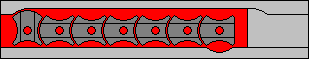Turn dials 1 to 6 horizontal, using the standard solution (first move is dial 2).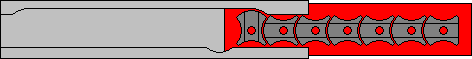Slide out the base as far as it will go.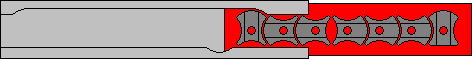You can now turn dial 1 vertical again, and then give dials 2, 3, and 4 a half turn each.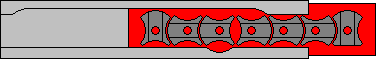Slide the base back in until dial 5 is at the spot in the frame where it can be turned.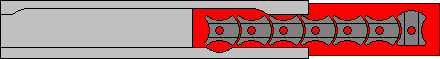Give dials 5 and 6 a half turn. Now dial 7 can also be turned horizontal, and the puzzle is solved.

This shortcut uses L6+7 = 42+7 = 49 moves instead of the usual 85. It can come in very handy if you started with the wrong move, ending up much further away from the solved position with six horizontal dials.
The Elephant Spinout has a slightly shorter frame, and you can apply this shortcut technique a little more easily since you only need to solve dials 1-5 in step a. This leads to a solution of L5+7=28 moves.
There is a cheap Taiwanese version (with dials of different colours) which is even shorter and needs only L4+7=17 moves!